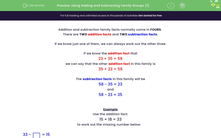# Find the Missing Number Using Addition and Subtraction Family Group Facts

In this worksheet, students will understand that addition and subtraction are inverses and use this fact to find the missing number.Key stage:  KS 1

Curriculum topic:   Number: Addition and Subtraction

Curriculum subtopic:   Understand Relationship Between Addition and Subtraction

Difficulty level:#### Worksheet Overview

This activity is all about families.No, not that sort of family -  maths families!

Addition and subtraction family facts normally come in fours

There are two addition facts and two subtraction facts.

If we know just one of them, we can always work out the other three.

If we know the addition fact that

23 + 35 = 58

we can say that the other addition fact in this family is

35 + 23 = 58

The subtraction facts in this family will be

58 - 35 = 23

and

58 - 23 = 35

Got that?Example

15 + 18 = 33

to work out the missing number below:

33 -= 15

15 + 18 = 33

So 33 - 18 = 15 and 33 - 15 = 18

The missing number is 18.

33 - 18  = 15

Remember that if you know just one of the family facts, you can work out all the other three.

Let's have a go at some questions now.

### What is EdPlace?

We're your National Curriculum aligned online education content provider helping each child succeed in English, maths and science from year 1 to GCSE. With an EdPlace account you’ll be able to track and measure progress, helping each child achieve their best. We build confidence and attainment by personalising each child’s learning at a level that suits them.

Get started